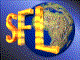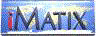| iMatix home page | << | < | > | >>SFLVersion 2.11

### tree_find_eq

```#include "sfltree.h"
void *tree_find_eq (TREE **root, void *tree, TREE_COMPARE *comp)
```

#### Synopsis

Finds a node with data exactly matching that provided.

#### Source Code - (sfltree.c)

```{
TREE
*current = *root,
*found;

found = NULL;
while (current != TREE_NULL)
switch ((comp) (current, tree))
{
case -1: current = current-> right; break;
case  1: current = current-> left;  break;
default: found = current;           /*  In case of duplicates,   */
current = current-> left;  /*  get the first one.       */
}

return found;
}
```

 | << | < | > | >>Copyright © 1996-2000 iMatix Corporation